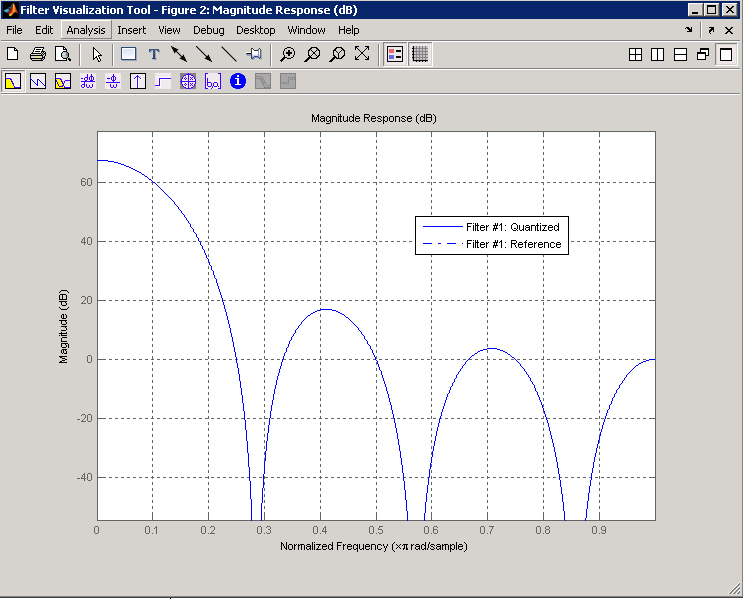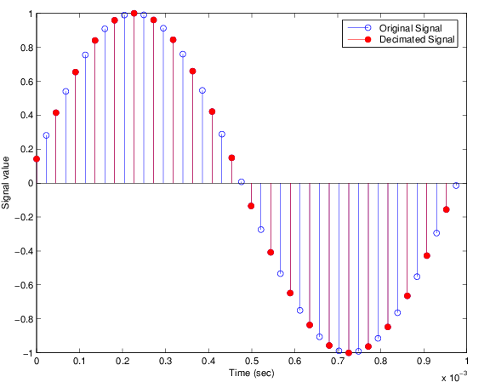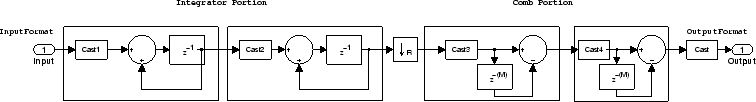# mfilt.cicdecim

Fixed-point CIC decimator

`mfilt.cicdecim` will be removed in a future release. Use `dsp.CICDecimator` instead.

## Syntax

```hm = mfilt.cicdecim(r,m,n,iwl,owl,wlps) ```

## Description

`hm = mfilt.cicdecim(r,m,n,iwl,owl,wlps)` returns a cascaded integrator-comb (CIC) decimation filter object.

All of the input arguments are optional. To enter any optional value, you must include all optional values to the left of your desired value.

When you omit one or more input options, the omitted option applies the default values shown in the table below.

The following table describes the input arguments for creating `hm`.

Input Arguments

Description

`r`

Decimation factor applied to the input signal. Sharpens the response curve to let you change the shape of the response. `r` must be an integer value greater than or equal to 1. The default value is 2.

`m`

Differential delay. Changes the shape, number, and location of nulls in the filter response. Increasing `m` increases the sharpness of the nulls and the response between nulls. In practice, differential delay values of 1 or 2 are the most common. `m` must be an integer value greater than or equal to 1. The default value is 1.

`n`

Number of sections. Deepens the nulls in the response curve. Note that this is the number of either comb or integrator sections, not the total section count. 2 is the default value.

`iwl`

Word length of the input signal. Use any integer number of bits. The default value is 16 bits.

`owl`

Word length of the output signal. It can be any positive integer number of bits. By default, `owl` is 16 bits.

`wlps`

Defines the number of bits per word in each filter section while accumulating the data in the integrator sections or while subtracting the data during the comb sections (using 'wrap' arithmetic). Enter `wlps` as a scalar or vector of length 2*`n`, where `n` is the number of sections. When `wlps` is a scalar, the scalar value is applied to each filter section. The default is 16 for each section in the decimator.

When you elect to specify `wlps` as an input argument, the `FilterInternals` property automatically switches from the default value of `'FullPrecision'` to `'SpecifyWordLengths'`.

### Constraints and Word Length Considerations

CIC decimators have the following constraint — the word lengths of the filter section must be monotonically decreasing. The word length of each filter section must be the same size as, or smaller than, the word length of the previous filter section.

The formula for Bmax, the most significant bit at the filter output, is given in the Hogenauer paper in the References below.

`${B}_{\mathrm{max}}=\left(N{\mathrm{log}}_{2}RM+{B}_{in}-1\right)$`

where Bin is the number of bits of the input.

The cast operations shown in the diagram in Algorithms perform the changes between the word lengths of each section. When you specify word lengths that do not follow the constraints above, the constructor returns an error.

When you specify the word lengths correctly, the most significant bit Bmax stays the same throughout the filter, while the word length of each section either decreases or stays the same. This can cause the fraction length to change throughout the filter as least significant bits are truncated to decrease the word length, as shown in Algorithms.

### Properties of the Object

Objects have properties that control the way the object behaves. This table lists all the properties for the filter, with a description of each.

Name

Values

Default

Description

`Arithmetic`

`fixed`

`fixed`

Reports the kind of arithmetic the filter uses. CIC decimators are always fixed-point filters.

`DecimationFactor`

Any positive integer

2

Amount to reduce the input sampling rate.

`DifferentialDelay`

Any positive integer

1

Sets the differential delay for the filter. Usually a value of one or two is appropriate.

`FilterStructure`

`mfilt` structure

None

Reports the type of filter object. You cannot set this property — it is always read only and results from your choice of `mfilt` objects.

`FilterInternals`

```FullPrecision, MinWordLengths, SpecifyPrecision, SpecifyWordLengths```

`FullPrecision`

Set the usage mode for the filter. Refer to Usage Modes below for details.

`InputFracLength`

Any positive integer

15

The number of bits applied to the fraction length to interpret the input data to the filter.

`InputOffset`

Integers in the range
0 ≤ `InputOffset` ≤ `r-1`

0

Contains a value derived from the number of input samples and the decimation factor — `InputOffset = mod(length(nx),m)` where `nx` is the number of input samples that have been processed so far and `m` is the decimation factor.

The `InputOffset` property applies only when you set the `PersistentMemory` property to `true`. See InputOffset for more information.

`InputWordLength`

Any positive integer

16

The number of bits applied to the word length to interpret the input data to the filter.

`NumberOfSections`

Any positive integer

2

Number of sections used in the decimator. Generally called `n`. Reflects either the number of decimator or comb sections, not the total number of sections in the filter.

`OutputFracLength`

Any positive integer

15

The number of bits applied to the fraction length to interpret the output data from the filter. Read-only.

`OutputWordLength`

Any positive integer

16

The number of bits applied to the word length to interpret the output data from the filter.

`PersistentMemory`

`false` or `true`

`false`

Determines whether the filter states get restored to their starting values for each filtering operation. The starting values are the values in place when you create the filter if you have not changed the filter since you constructed it. `PersistentMemory` returns to zero any state that the filter changes during processing. States that the filter does not change are not affected. When `PersistentMemory` is `false`, you cannot access the filter states. Setting `PersistentMemory` to `true` reveals the `States` property so you can modify the filter states.

NameValuesDefaultDescription

```SectionWord  Lengths```

Any integer or a vector of length 2*`n`.

16

Defines the bits per section used while accumulating the data in the integrator sections or while subtracting the data during the comb sections (using 'wrap' arithmetic). Enter `SectionWordLengths` as a scalar or vector of length 2*`n`, where `n` is the number of sections. When `SectionWordLengths` is a scalar, the scalar value is applied to each filter section. When `SectionWordLengths` is a vector of values, the values apply to the sections in order. The default is 16 for each section in the decimator. Available when `FilterInternals` is `'SpecifyWordLengths'`.

`States`

`filtstates.cic` object

`m`+1-by-`n` matrix of zeros, after you call function `int`.

Stored conditions for the filter, including values for the integrator and comb sections before and after filtering. `m` is the differential delay of the comb section and `n` is the number of sections in the filter. The integrator states are stored in the first matrix row. States for the comb section fill the remaining rows in the matrix. Available for modification when `PersistentMemory` is `true`. Refer to the `filtstates` object in Signal Processing Toolbox™ documentation for more general information about the `filtstates` object.

### Usage Modes

There are four modes of usage for this which are set using the `FilterInternals` property

• `FullPrecision` — All word and fraction lengths set to Bmax + 1, called Baccum by Fred Harris in . Full Precision is the default setting.

• `MinWordLengths` — Automatically set the sections for minimum word lengths.

• `SpecifyWordLengths` — Specify the word lengths for each section.

• `SpecifyPrecision` — Specify precision by providing values for the word and fraction lengths for each section.

Full Precision

In full precision mode, the word lengths of all sections and the output are set to Baccum as defined by

`${B}_{accum}=ceil\left({N}_{\mathrm{sec}s}\left(Lo{g}_{2}\left(D×M\right)\right)+InputWordLength\right)$`

where Nsecs is the number of filter sections.

Section fraction lengths and the fraction length of the output are set to the input fraction length.

Here is the display for this mode:

```FilterStructure: 'Cascaded Integrator-Comb Decimator' Arithmetic: 'fixed' DifferentialDelay: 1 NumberOfSections: 2 DecimationFactor: 4 PersistentMemory: false InputWordLength: 16 InputFracLength: 15 FilterInternals: 'FullPrecision'```

Minimum Wordlengths

In minimum word length mode, you control the output word length explicitly. When the output word length is less than Baccum, roundoff noise is introduced at the output of the filter. Hogenauer's bit pruning theory (refer to ) states that one valid design criterion is to make the word lengths of the different sections of the filter smaller than Baccum as well, so that the roundoff noise introduced by all sections does not exceed the roundoff noise introduced at the output.

In this mode, the design calculates the word lengths of each section to meet the Hogenauer criterion. The algorithm subtracts the number of bits computed using eq. 21 in Hogenauer's paper from Baccum to determine the word length each section.

To compute the fraction lengths of the different sections, the algorithm notes that the bits thrown out for this word length criterion are least significant bits (LSB), therefore each bit thrown out at a particular section decrements the fraction length of that section by one bit compared to the input fraction length. Setting the output wordlength for the filter automatically sets the output fraction length as well.

Here is the display for this mode:

```FilterStructure: 'Cascaded Integrator-Comb Decimator' Arithmetic: 'fixed' DifferentialDelay: 1 NumberOfSections: 2 DecimationFactor: 4 PersistentMemory: false InputWordLength: 16 InputFracLength: 15 FilterInternals: 'MinWordLengths' OutputWordLength: 16```

Specify word lengths

In this mode, the design algorithm discards the LSBs, adjusting the fraction length so that unrecoverable overflow does not occur, always producing a reasonable output.

You can specify the word lengths for all sections and the output, but you cannot control the fraction lengths for those quantities.

To specify the word lengths, you enter a vector of length 2*(`NumberOfSections`), where each vector element represents the word length for a section. If you specify a scalar, such as Baccum, the full-precision output word length, the algorithm expands that scalar to a vector of the appropriate size, applying the scalar value to each section.

The CIC design does not check that the specified word lengths are monotonically decreasing. There are some cases where the word lengths are not necessarily monotonically decreasing, for example

```hcic=mfilt.cicdecim; hcic.FilterInternals='minwordlengths'; hcic.Outputwordlength=14;```

which are valid CIC filters but the word lengths do not decrease monotonically across the sections.

Here is the display for the `SpecifyWordLengths` mode.

```FilterStructure: 'Cascaded Integrator-Comb Decimator' Arithmetic: 'fixed' DifferentialDelay: 1 NumberOfSections: 2 DecimationFactor: 4 PersistentMemory: false InputWordLength: 16 InputFracLength: 15 FilterInternals: 'SpecifyWordLengths' SectionWordLengths: [19 18 18 17] OutputWordLength: 16```

Specify precision

In this mode, you have full control over the word length and fraction lengths of all sections and the filter output.

When you elect the `SpecifyPrecision` mode, you must enter a vector of length 2*(`NumberOfSections`) with elements that represent the word length for each section. When you enter a scalar such as Baccum, `mfilt.cicdecim` expands that scalar to a vector of the appropriate size and applies the scalar value to each section and the output. The design does not check that this vector is monotonically decreasing.

Also, you must enter a vector of length 2*(`NumberOfSections`) with elements that represent the fraction length for each section as well. When you enter a scalar such as Baccum, `mfilt.cicdecim` applies scalar expansion as done for the word lengths.

Here is the `SpecifyPrecision` display.

```FilterStructure: 'Cascaded Integrator-Comb Decimator' Arithmetic: 'fixed' DifferentialDelay: 1 NumberOfSections: 2 DecimationFactor: 4 PersistentMemory: false InputWordLength: 16 InputFracLength: 15 FilterInternals: 'SpecifyPrecision' SectionWordLengths: [19 18 18 17] SectionFracLengths: [14 13 13 12] OutputWordLength: 16 OutputFracLength: 11 ```

### About the States of the Filter

In the `states` property you find the states for both the integrator and comb portions of the filter. `states` is a matrix of dimensions `m` + 1-by-`n`, with the states apportioned as follows:

• States for the integrator portion of the filter are stored in the first row of the state matrix.

• States for the comb portion fill the remaining rows in the state matrix.

To review the states of a CIC filter, use `int` to assign the states to a variable in MATLAB. As an example, here are the states for a CIC decimator `hm` before and after filtering a data set.

```x = fi(ones(1,10),true,16,0); % Fixed-point input data. hm = mfilt.cicdecim(2,1,2,16,16,16); sts=int(hm.states) set(hm,'InputFracLength',0); % Integer input specified. y=filter(hm,x); sts=int(hm.states) ```

STS is an integer matrix that `int` returns from the contents of the `filtstates.cic` object in `hm`.

### Design Considerations

When you design your CIC decimation filter, remember the following general points:

• The filter output spectrum has nulls at ω = k * 2π/`rm` radians, k = 1,2,3....

• Aliasing and imaging occur in the vicinity of the nulls.

• `n`, the number of sections in the filter, determines the passband attenuation. Increasing `n` improves the filter ability to reject aliasing and imaging, but it also increases the droop (or rolloff) in the filter passband. Using an appropriate FIR filter in series after the CIC decimation filter can help you compensate for the induced droop.

• The DC gain for the filter is a function of the decimation factor. Raising the decimation factor increases the DC gain.

## Examples

This example applies a decimation factor `r` equal to `8` to a 160-point impulse signal. The signal output from the filter has 160/`r`, or 20, points or samples. Choosing 10 bits for the word length represents a fairly common setting for analog to digital converters. The plot shown after the code presents the stem plot of the decimated signal, with 20 samples remaining after decimation:

```m = 2; % Differential delays in the filter. n = 4; % Filter sections r = 8; % Decimation factor x = int16(zeros(160,1)); x(1) = 1; % Create a 160-point % impulse signal. hm = mfilt.cicdecim(r,m,n); % Expects 16-bit input % by default. y = filter(hm,x); stem(double(y)); % Plot output as a stem plot. xlabel('Samples'); ylabel('Amplitude'); title('Decimated Signal');```The next example demonstrates one way to compute the filter frequency response, using a 4-section decimation filter with the decimation factor set to `7`:

```hm = mfilt.cicdecim(7,1,4); fvtool(hm)```

FVTool provides ways for you to change the title and x labels to match the figure shown. Here's the frequency response plot for the filter. For details about the transfer function used to produce the frequency response, refer to  in the References section.This final example demonstrates the decimator for converting from 44.1 kHz audio to 22.05 kHz — decimation by two. To overlay the before and after signals, scale the output and plot the signals on a stem plot.

```r = 2; % Decimation factor. hm = mfilt.cicdecim(r); % Use default NumberOfSections & % DifferentialDelay property values. fs = 44.1e3; % Original sampling frequency: 44.1kHz. n = 0:10239; % 10240 samples, 0.232 second long signal. x = sin(2*pi*1e3/fs*n);% Original signal, sinusoid at 1kHz. y_fi = filter(hm,x); % 5120 samples, still 0.232 seconds. % Scale the output to overlay the stem plots. x = double(x); y = double(y_fi); y = y/max(abs(y)); stem(n(1:44)/fs,x(2:45)); hold on; % Plot original signal % sampled at 44.1kHz. stem(n(1:22)/(fs/r),y(3:24),'r','filled'); % Plot decimated % signal (22.05kHz) % in red. xlabel('Time (seconds)');ylabel('Signal Value');```## Algorithms

To show how the CIC decimation filter is constructed, the following figure presents a block diagram of the filter structure for a two-section CIC decimation filter (`n` = 2). fs is the high sampling rate, the input to the decimation process.

For details about the bits that are removed in the Comb section, refer to  in References.`mfilt.cicdecim` calculates the fraction length at each section of the decimator to avoid overflows at the output of the filter.

## References

 Hogenauer, E. B., “An Economical Class of Digital Filters for Decimation and Interpolation,” IEEE® Transactions on Acoustics, Speech, and Signal Processing, ASSP-29(2): pp. 155-162, 1981

 Meyer-Baese, Uwe, “Hogenauer CIC Filters,” in Digital Signal Processing with Field Programmable Gate Arrays, Springer, 2001, pp. 155-172

 Harris, Fredric J, Multirate Signal Processing for Communication Systems, Prentice-Hall PTR, 2004 , pp. 343